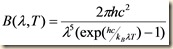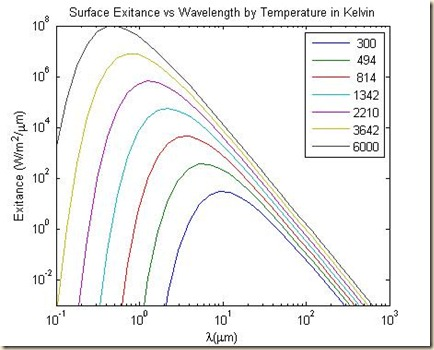## Monday, July 16, 2012

### Radiometry Calculation Error, Part I: Background

No discussion of radiometry would be complete without Planck’s equation, which describes the power of the radiation emitted from a surface at a given temperature.  As a function of wavelength λ and temperature T in Kelvin, Planck’s equation is:where h is Planck’s constant, kB is Boltzmann’s constant, and c is the speed of light in a vacuum.

Below is the iconic graph of Planck’s equation in units of Watts per square meter per micron for wavelengths ranging from the UV through the thermal towards the RF, and temperatures ranging from room temperature (300K) to the sun’s surface (6000K).  We note that as the temperature increases, the peak of the curve shifts towards the shorter wavelengths.  For instance, the strength of the sun’s radiation conveniently peaks in the visible wavelengths (roughly 0.4 – 0.7 microns).  While thermal emissions are quite strong (human eyes are sensitive to about 10-3 Watts/m2), they are only strong in the thermal wavelengths (7 – 20 microns), beyond where we can see.I should also note that in practice any radiation sensor will collect over a finite bandpass; thus, Planck’s equation must be integrated over whatever wavelengths are under consideration.  In this respect, Planck’s equation is much like a probability density function, which must also be integrated over a range of values to find their probability.

Of course, knowing the surface exitance alone doesn’t tell us the radiation density reaching a given point, but before we discuss this, we should define some terms:

• Exitance (M):  the power density emitted by a surface, measured in Watts / m2.  We will reserve B for the per-micron un-integrated quantity.
• Radiance (L):  the power density emitted by a surface into each solid angle, measured in Watts / m2 / steradian.
• Intensity (I):  the power emitted by a point source into each solid angle, measured in Watts / steradian.
• Irradiance (E):  the power incident upon a receiving surface, measured in Watts / m2.

For those of you without much background in solid geometry, I will discuss solid angles in the next lesson, but for now consider your own experience.  We have little trouble seeing most objects without regard to either the angle of observation or the angle of the incident light.  This is because the object is reflecting most of the light equally in all directions into the surrounding hemisphere.  This kind of reflection is called lambertian.  On the other hand, if you hold the object up to the light, most of the time you can find an angle at which you see a glint or bright spot; this kind of reflection is called specular.  In the case of a mirror, for instance, almost all the incident light is reflected specularly.  An object’s particular mix of lambertian and specular behavior is called its bidirectional reflectance distribution function (BRDF); however, absent this specific knowledge, the lambertian assumption is usually the safest, especially for emitted as opposed to reflected radiation.

I will discuss the relationship between these quantities in my next lesson.